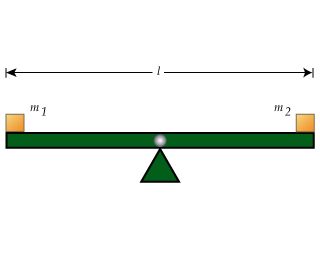# Problem: Learning Goal:To understand and apply the formula τ = Iα to rigid objects rotating about a fixed axis.To find the acceleration a of a particle of mass m, we use Newton's second law: Fnet = ma, where Fnet is the net force acting on the particle.To find the angular acceleration α of a rigid object rotating about a fixed axis, we can use a similar formula: τnet = Iα, where τnet =∑τ is the net torque acting on the object and I is its moment of inertia.In this problem, you will practice applying this formula to several situations involving angular acceleration. In all of these situations, two objects of masses m1 and m2 are attached to a seesaw. The seesaw is made of a bar that has length l and is pivoted so that it is free to rotate in the vertical plane without friction. Assume that the pivot is attached to The center of the bar.You are to find the angular acceleration of the seesaw when it is set in motion from the horizontal position. In all cases, assume that m1 &gt; m2.Part ANow consider a similar situation, except that now the swing bar itself has mass mbar.(Figure 2)Find the magnitude of the angular acceleration α of the seesaw.Express your answer in terms of some or all of the quantities m1, m2, mbar, l, as well as the acceleration due to gravity g.Part BIn what direction will the seesaw rotate and what will the sign of the angular acceleration be?A. The rotation is in the clockwise direction and the angular acceleration is positive.B. The rotation is in the clockwise direction and the angular acceleration is negative.C. The rotation is in the counterclockwise direction and the angular acceleration is positive.D. The rotation is in the counterclockwise direction and the angular acceleration is negative.

###### FREE Expert Solution

Torque equations:

$\overline{){\mathbf{\Sigma }}{\mathbf{\tau }}{\mathbf{=}}{\mathbf{\alpha }}{\mathbf{I}}}$ where I is the moment of inertia and α is the angular acceleration.

$\overline{){\mathbit{\tau }}{\mathbf{=}}{\mathbit{r}}{\mathbit{F}}}$

Moment of inertia of a point mass:

$\overline{){\mathbf{I}}{\mathbf{=}}{\mathbf{m}}{{\mathbf{r}}}^{{\mathbf{2}}}}$

Moment of inertia of a rod about its center of mass:

$\overline{){\mathbf{I}}{\mathbf{=}}\frac{\mathbf{1}}{\mathbf{12}}{\mathbf{M}}{{\mathbf{L}}}^{{\mathbf{2}}}}$

(a)

I = m1(l/2)2 + m2(l/2)2 + (1/12)mbarl2 = (m1 + m2 + mbar/3)l2/4

Στ  = Iα

89% (232 ratings)###### Problem Details

Learning Goal:

To understand and apply the formula τ = Iα to rigid objects rotating about a fixed axis.

To find the acceleration a of a particle of mass m, we use Newton's second law: Fnet = ma, where Fnet is the net force acting on the particle.

To find the angular acceleration α of a rigid object rotating about a fixed axis, we can use a similar formula: τnet = Iα, where τnet =∑τ is the net torque acting on the object and I is its moment of inertia.In this problem, you will practice applying this formula to several situations involving angular acceleration. In all of these situations, two objects of masses m1 and m2 are attached to a seesaw. The seesaw is made of a bar that has length l and is pivoted so that it is free to rotate in the vertical plane without friction. Assume that the pivot is attached to The center of the bar.

You are to find the angular acceleration of the seesaw when it is set in motion from the horizontal position. In all cases, assume that m1 > m2.

Part A

Now consider a similar situation, except that now the swing bar itself has mass mbar.(Figure 2)

Find the magnitude of the angular acceleration α of the seesaw.

Express your answer in terms of some or all of the quantities m1, m2, mbar, l, as well as the acceleration due to gravity g.

Part B

In what direction will the seesaw rotate and what will the sign of the angular acceleration be?

A. The rotation is in the clockwise direction and the angular acceleration is positive.
B. The rotation is in the clockwise direction and the angular acceleration is negative.
C. The rotation is in the counterclockwise direction and the angular acceleration is positive.
D. The rotation is in the counterclockwise direction and the angular acceleration is negative.